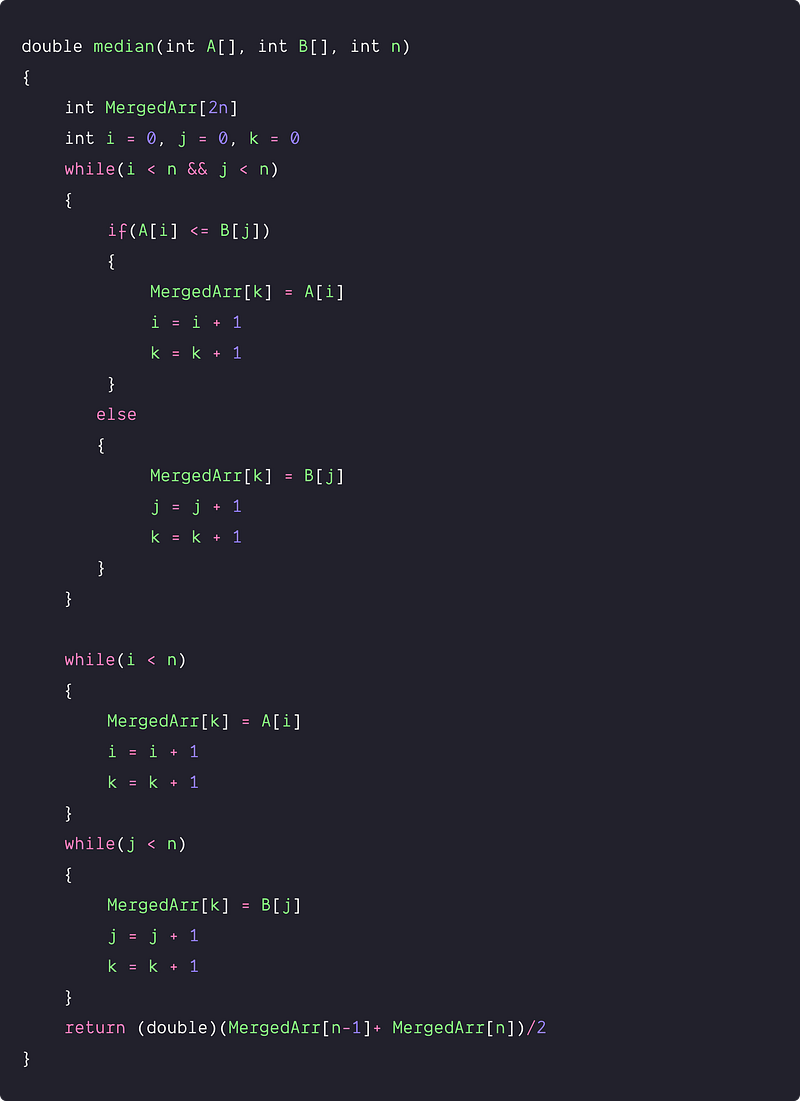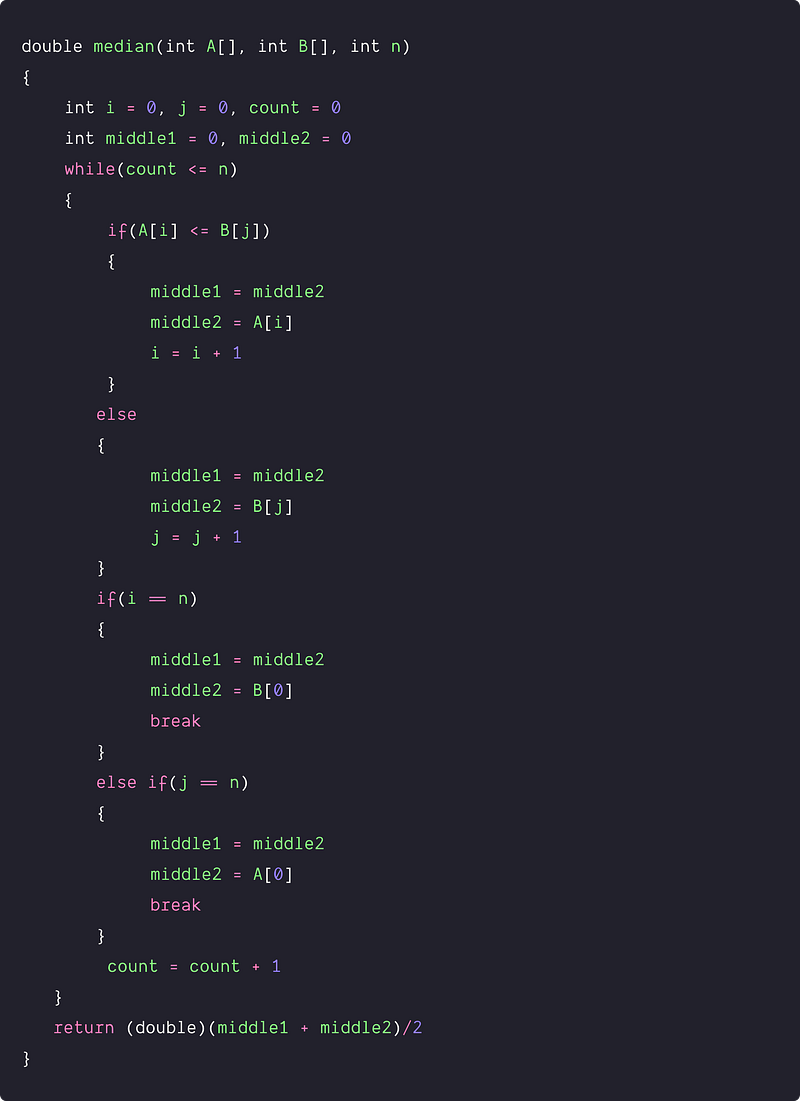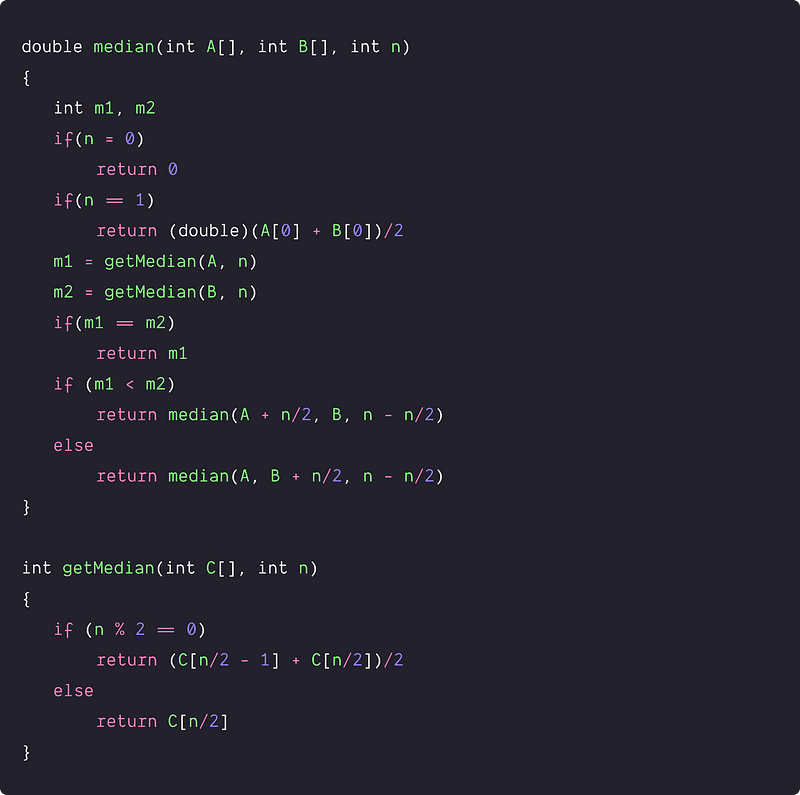# Median of two sorted arrays of the equal size

Key takeaway: An excellent problem to learn problem-solving using two pointers idea similar to merging and divide and conquer idea similar to the binary search.

### Let's understand the problem

There are two sorted arrays A[] and B[] of size n each, write a program to find the median of the array obtained after merging both the arrays i.e. merged array of size 2n.

• The median of a sorted array of size n is defined as the middle element when n is odd and the average of the middle two elements when n is even.
• After merging both arrays, the size of the larger array will be 2n i.e. an even value.
• For the convenience of the solution, let's assume n is odd.

Example 1

Input: A[] = [1, 3, 6], B[] = [2, 8, 12]

Output: 4.5

Explanation: After merging sorted arrays, we get the larger sorted array [1, 2, 3, 6, 8, 12]. The total number of elements is 6, so the median would be the average of the two middle elements at index 2 and 3 i.e. (3 + 6)/2 = 4.5

Example 2

Input: A[] = [1, 3, 4, 6, 9], B[] = [2, 5, 7, 8, 10]

Output: 5.5

Explanation: After merging sorted arrays, we get the larger sorted array [1, 2, 3, 4, 5, 6, 7, 8, 9, 10]. So median would be the average of the two middle elements at index 4 and 5 i.e. (5 + 6)/2 = 5.5

### Discussed solution approaches

• Brute force approach: merging using extra space
• Two-pointers approach: counting while merging
• Efficient approach : divide and conquer idea similar to the binary search

### Brute force approach: merging using extra space

Solution Idea

So one basic idea is to merge both sorted arrays using extra space and get the median by taking an average of both middle elements in the merged array. If we follow the 0 based indexing, the median will be the average value at (n-1)th and nth indexes. Note: for merging sorted arrays, we can use an idea similar to the merging procedure of the merge sort.

Solution PseudocodeTime and space complexity analysis

• We need to traverse each element to merge both sorted arrays. In other words, at each iteration of while loops, we place one value to the MergedArr[]. So time complexity of merging = O(n)
• Overall time complexity = Time complexity of merging + Time complexity of finding median = O(n) + O(1) = O(n)
• We are using 2n size extra space for merging, so space complexity = O(n)

### Two-pointers approach: Counting while merging

Solution Idea

Now critical questions are: do we really need to perform the complete merging of sorted arrays to find the median? can we reduce the extra space used in the merging process? Let's Think!

Here are few critical observations: we are traversing both the arrays from start in incremental order. At each step of the iteration, we are increasing either pointer i or pointer j and placing one value from any one of the smaller arrays to the larger sorted array. At any general step of iteration, k = i + j - 1.

So here is an optimized solution idea: while comparing the elements of two sorted arrays during the merging process, we can track the count of elements in the merged array using variable k. We stop when the count becomes n + 1 and calculate the median by taking an average of the nth and (n+1)th element of the merged array. So there is no need to use extra space and perform complete merging.

Solution Steps

1. We initialize two pointers i and j to move in A[] and B[]. i = 0 and j = 0.
2. We also initialize three variables: middle1 and middle2 to keep track of the middle elements and count to track the count of elements in the merged array. middle1 = 0, middle2 = 0 and count =0.
3. Now we start the merging loop and move forward by comparing the elements in A[] and B[] until the count becomes equal to the n +1. We also keep track of the middle elements middle1 and middle2.
4. By end of the loop, we return the median by taking an average of the middle1 and middle2.

Solution PseudocodeTime and Space complexity analysis

While loop is running n times and doing O(1) operation at each iteration. So the time complexity = n * O(1) = O(n). We are using a constant number of extra variables, so space complexity = O(1).

### Efficient approach : divide and conquer idea similar to the binary search

Now critical questions are: how do we improve the time complexity? This is a searching problem, so can we take advantage of the sorted array property? Let's think!

Solution Idea

Here idea is to compare the medians of both sorted arrays and recursively reduce the search space by half. Suppose the median of the first array is m1, and the second array is m2. We can get this value in O(1) using the formula: m1 = A[n/2], m2 = B[n/2] (We have assumed in the problem that n is odd)

Case 1 if (m1 = m2): In this case, n-1 elements are less than m1 and n-1 elements are greater than m2. In other words, both m1 and m2 would be the middle elements in the merged array i.e. element at (n-1)th and nth index. We take the average of both, which is equal to m1 or m2. We return any one of the values as an output. (Think!)

Case 2 if (m1 < m2): then in the merged array, both middle elements of the median will lie between the range [m1, m2] i.e. m1 <= (middle1, middle2) <= m2.

• We can ignore the left half of the array A[]: all the values less than m1 will always be the starting elements in the merged array (n/2 values, if n is odd) i.e. somewhere present in the range of index from 0 to n-2 in the merged array (think!). In other words, these values will never be middle values in the merged array. So, we ignore the left half of array A[] from index 0 to n/2 - 1.
• We can ignore the right half of the array B[]: all the values greater than m2 will always be the ending elements in the merged array (n/2 values if n is odd) i.e. somewhere present in the range of index from n+1 to 2n-1 in the merged array (think!). In other words, these values will never be middle values in the merged array. So, we ignore the right half of array B[] from index n/2 + 1 to n - 1.
• In other words, both middle values of the median will be present in one of the two subarrays: right half of array A[] and left half of array B[].

Case 3 if (m1 > m2): then in the merged array, both middle elements of the median will lie between the range [m2, m1] i.e. m2 <= (middle1, middle2) <= m1.

• We can ignore the right half of the array A[]: all the values greater than m1 will always be the ending elements in the merged array (n/2 values if n is odd) i.e. somewhere present in the range of index from n+1 to 2n-1 in the merged array (think!). In other words, these values will never be middle values in the merged array. So, we ignore the right half of array A[] from index n/2 + 1 to n-1.
• We can ignore the left half of the array B[]: all the values less than m2 will always be the starting elements in the merged sorted array (n/2 values if n is odd) i.e. somewhere present in the range of index from 0 to n-2 in the merged array (think!). In other words, these values will never be middle values in the merged array. So, we ignore the left half of array B[] from index 0 to n/2 -1.
• In other words, both middle values of the median will be present in one of the two subarrays: left half of array A[] and right half of array B[].

The above idea is quite similar to the binary search: we reject half elements in both sorted arrays after each comparison. Overall, we are reducing the search space by half after each step. So, we can think of implementing a solution using an idea similar to a recursive binary search.

Solution Steps

Suppose we use function median(int A[], int B[], int n).

• We first find the medians m1 and m2 of the arrays A[] and B[]. If the value of m1 is equal to m2, then we return m1 or m2.
• If (m1 > m2), then both middle elements of the median must be present in one of the two sub-arrays: subarray starting from the first element of array A[] and ending at element m1(including m1) and subarray starting from element m2 (including m2) and ending at the last element of array B[]. In other words, we are rejecting n/2 elements from both subarrays and the remaining input size = n - n/2. So, we recursively call the same function with input size n - n/2 i.e. median(A, B + n/2, n - n/2)

For example, if n = 7, then n/2 = 3, m1 = A and m2 = B. So we reject: 3 elements in A[] from index 4 to 6 and 3 elements in B[] from index 0 to 2. Remaining size of array A and B = n - n/2 = 7 - 3 = 4.

• If (m2 > m1), both middle elements of the median must be present in one of the two sub-arrays: subarray starting from element m1 (including m1) and ending at the last element of array A[] and subarray starting from the first element of array B[] and ending at element m2 (including m2). In other words, we are rejecting n/2 elements from both subarrays and the remaining input size = n - n/2. So, we recursively call the same function with input size n - n/2 i.e. median(A + n/2, B, n - n/2)

For example, if n = 9, then n/2 = 4, m1 = A and m2 = B. So we reject: 4 elements in A[] from index 0 to 3, and 4 elements in B[] from index 5 to 8. Remaining size of array A and B = n - n/2 = 9 - 4 = 5.

• Base case: here n is odd, so base occur when n <=1. if (n == 0), we return 0, and if(n == 1), we return the average of A and B.

Solution PseudocodeTime and space complexity analysis

• Input size given in the problem = Size of A[] + Size of B[] = 2n . At each step of recursion, we are reducing the input size by 1/2 i.e. we are rejecting one-half of both the smaller arrays or reducing the search space by half.
• Recurrence relation, T(2n) = T(n) + O(1), or we can write T(x) = T(x/2) + O(1), where x = 2n. This looks similar to the recurrence relation of the binary search. So, Time Complexity = O(log n).
• Space Complexity = O(log n), which is equal to the size of the recursion call stack (Think!)

### Critical ideas to think!

• Can we solve this problem in O(logn) time complexity using an iterative approach?
• How do we modify the above algorithms when n is even?
• How do we modify the above algorithms when n can be both odd or even?
• How can we solve the above algorithms if the sizes of both arrays are different? What would be the time complexity? What are the boundary conditions?
• In the 2nd approach, why are there two different boundary cases for i = n and j = n?
• In the second approach, why are we including m1 and m2 during the recursive call?
• Visualize and dry run the effcient approach.

### Comparison of time and space complexities

• Merging using extra space: Time = O(n), Space = O(n)
• Using a two-pointer approach: Time = O(n), Space = O(1)
• Using divide and conquer approach: Time = O(logn), Space = O(logn)

### Suggested coding problems to practice

• Inversion count in an array
• Median of two sorted arrays of different sizes
• K-th Element of Two Sorted Arrays
• Josephus problem
• Find median in the row-wise sorted matrix
• Merge two sorted arrays

Enjoy learning, Enjoy algorithms!

Get well-designed application and interview centirc content on ds-algorithms, machine learning, system design and oops. Content will be delivered weekly.